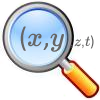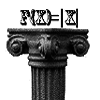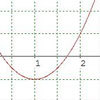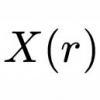Search by Topic

Resources tagged with Calculus generally similar to Scientific Curves:

Filter by: Content type:
Age range:
Challenge level:

There are 27 results

Broad Topics > Calculus > Calculus generallyScientific Curves

Age 16 to 18 Challenge Level:

Can you sketch these difficult curves, which have uses in mathematical modelling?Population Dynamics Collection

Age 16 to 18 Challenge Level:

This is our collection of tasks on the mathematical theme of 'Population Dynamics' for advanced students and those interested in mathematical modelling.Patterns of Inflection

Age 16 to 18 Challenge Level:

Find the relationship between the locations of points of inflection, maxima and minima of functions.Calculus Analogies

Age 16 to 18 Challenge Level:

Consider these analogies for helping to understand key concepts in calculus.Population Ecology Using Probability

Age 16 to 18 Challenge Level:

An advanced mathematical exploration supporting our series of articles on population dynamics for advanced students.Population Dynamics - Part 6

Age 16 to 18 Challenge Level:

Sixth in our series of problems on population dynamics for advanced students.Population Dynamics - Part 5

Age 16 to 18 Challenge Level:

Fifth in our series of problems on population dynamics for advanced students.Population Dynamics - Part 4

Age 16 to 18 Challenge Level:

Fourth in our series of problems on population dynamics for advanced students.Population Dynamics - Part 3

Age 16 to 18 Challenge Level:

Third in our series of problems on population dynamics for advanced students.Population Dynamics - Part 2

Age 16 to 18 Challenge Level:

Second in our series of problems on population dynamics for advanced students.Population Dynamics - Part 1

Age 16 to 18 Challenge Level:

First in our series of problems on population dynamics for advanced students.A Different Differential Equation

Age 16 to 18 Challenge Level:

Explore the properties of this different sort of differential equation.Branching Processes and Extinction

Age 16 to 18 Challenge Level:

An advanced mathematical exploration supporting our series of articles on population dynamics for advanced students.Integral Arranging

Age 16 to 18 Challenge Level:

How would you sort out these integrals?Maths Shop Window

Age 16 to 18 Challenge Level:

Make a functional window display which will both satisfy the manager and make sense to the shoppersCurvy Catalogue

Age 16 to 18 Challenge Level:

Make a catalogue of curves with various properties.Fractional Calculus I

Age 16 to 18

You can differentiate and integrate n times but what if n is not a whole number? This generalisation of calculus was introduced and discussed on askNRICH by some school students.Interactive Workout - Mathmo

Age 16 to 18 Short Challenge Level:

Mathmo is a revision tool for post-16 mathematics. It's great installed as a smartphone app, but it works well in pads and desktops and notebooks too. Give yourself a mathematical workout!Turning to Calculus

Age 16 to 18 Challenge Level:

Get started with calculus by exploring the connections between the sign of a curve and the sign of its gradient.Operating Machines

Age 16 to 18 Challenge Level:

What functions can you make using the function machines RECIPROCAL and PRODUCT and the operator machines DIFF and INT?Implicitly

Age 16 to 18 Challenge Level:

Can you find the maximum value of the curve defined by this expression?Fractional Calculus II

Age 16 to 18

Here explore some ideas of how the definitions and methods of calculus change if you integrate or differentiate n times when n is not a whole number.Age 16 to 18 Challenge Level:

Can you find a quadratic equation which passes close to these points?Calculus Countdown

Age 16 to 18 Challenge Level:

Can you hit the target functions using a set of input functions and a little calculus and algebra?Fractional Calculus III

Age 16 to 18

Fractional calculus is a generalisation of ordinary calculus where you can differentiate n times when n is not a whole number.2D-3D

Age 16 to 18 Challenge Level:

Two circles of equal size intersect and the centre of each circle is on the circumference of the other. What is the area of the intersection? Now imagine that the diagram represents two spheres of. . . .Power Up

Age 16 to 18 Challenge Level:

Show without recourse to any calculating aid that 7^{1/2} + 7^{1/3} + 7^{1/4} < 7 and 4^{1/2} + 4^{1/3} + 4^{1/4} > 4 . Sketch the graph of f(x) = x^{1/2} + x^{1/3} + x^{1/4} -x